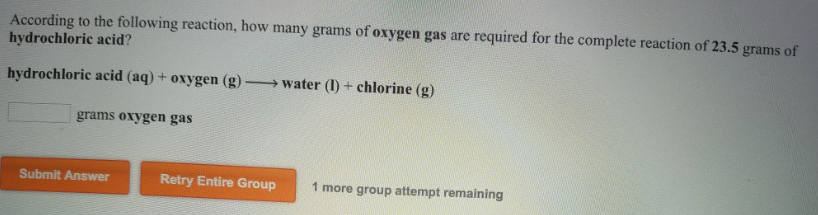# Problem: According to the following reaction, how many grams of oxygen gas are required for the complete reaction of 23.5 grams of hydrochloric acid? hydrochloric acid (aq) + oxygen (g) → water (l) + chlorine (g)

🤓 Based on our data, we think this question is relevant for Professor Yang's class at UT.

###### FREE Expert Solution###### Problem Details

According to the following reaction, how many grams of oxygen gas are required for the complete reaction of 23.5 grams of hydrochloric acid?

hydrochloric acid (aq) + oxygen (g) → water (l) + chlorine (g)